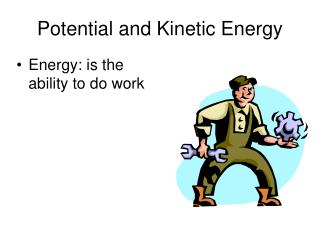DownloadDownload PresentationPotential and Kinetic Energy

# Potential and Kinetic Energy

Download Presentation## Potential and Kinetic Energy

- - - - - - - - - - - - - - - - - - - - - - - - - - - E N D - - - - - - - - - - - - - - - - - - - - - - - - - - -
##### Presentation Transcript

1. Potential and Kinetic Energy • Energy: is the ability to do work

2. Kinetic Energy= the energy an object possesses because of its motion • The amount of Kinetic energy is dependent on the mass of the object in motion and it’s velocity.

3. VELOCITY Velocity = distance ÷ time Distance = meters Time = seconds

4. Calculating kinetic energy If we know the mass of an object and its velocity we can determine the amount of kinetic energy possessed by using the following formula: kinetic energy = 1/2 (mass of object)(velocity of object)2 or KE = 1/2 mv2 or KE = 0.5mv2 The SI unit for kinetic energy is the Joule (J). A Joule is kg  m2/s2

5. A bicycle with a mass of 14 kg traveling at a velocity of 3.0 m/s east has how much kinetic energy? KE = 0.5mv2 = 0.5(14 kg)(3.0 m/s)2 = 0.5(14 kg)(9.0 m2/s2) = 63 kg x m2/s2 = 63 J

6. A 1200 kg automobile is traveling at a velocity of 100 m/s northwest. What is the kinetic energy of the automobile? KE = 0.5 mv2 = 0.5(1200kg)(100 m/s)2 = 0.5(1200 kg)(104 m2/s2) = 6 x 106 kg  m2/s2 = 6 x 106 J

7. Potential Energy • The energy of position • The amount of energy contained in an object at rest

8. Determining Potential Energy • By its position and its weight (mass X gravity) PE = (mass)(gravity)(height) = mgh • where m is mass in kg • g is the force of gravity = 9.8 m/s2 • h is the height • The SI unit that represents potential energy is the Joule (J) (kg  m2/s2).

9. Examine an example of potential energy A flower pot with a mass of 15 kg is sitting on a window sill 15 meters above the ground. How much potential energy does the flower pot contain? • PE = (mass)(gravity)(height) • = (15 kg)(9.8 m/s2)(15 m) • = 2205 kg  m2/s2 • = 2205 J • = 2.2 x 103J

10. Examine an example of potential energy • If the flower pot is lowered to a window sill that is 10. m from the ground. Does this change the potential energy of the flower pot? PE = (mass)(gravity)(height) = (15 kg)(9.8 m/s2)(10. m) = 1470 kg  m2/ = 1470 J = 1.5 x 103J

11. Potential or Kinetic Energy? • Moving car • Tree branch • Bent car fender • Balloon filled with air • Balloon squirting around room • Person inside a moving car

12. What type of energy does the space shuttle have at lift off?

13. Conversion of Potential to Kinetic Energy • In this picture both kinds of energy are evident. Can you point them out?

14. The water at the top has potential energy • When water falls to a lower level, the potential energy is converted to kinetic energy.

15. Example